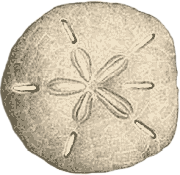Draw a pentagon or star using only a straightedge and compass

Ptolemy’s (extremely simple) method:

1.  Draw a circle on a horizontal line.2.  Bisect the radius $$\linesegment{OR}$$ at A.

3.  Add a vertical segment $$\linesegment{OB}$$ through the center.

4.  Draw the arcthrough the top of the circle B centered on A.

5.  The segment $$\linesegment{BC}$$ is the length of a side of an inscribed pentagon; use it to mark the vertices on the circle.

( Side note:  $$\linesegment{CO}$$ : $$\linesegment{OR}$$ = $$\linesegment{OR}$$ : $$\linesegment{CR}$$ = the golden ratio. )

Proof that the method is correct:

$$\linesegment{AB} = \sqrt{ 1^2 + \left(\frac{1}{2} \right)^2 } = \frac{\sqrt{5}}{2}$$

$$\linesegment{BC} = \sqrt{ 1^2 + \left(\linesegment{AB} - \frac{1}{2} \right)^2 } = \sqrt{ \smash{ \frac{ {5} - \sqrt{5} }{2} } \vphantom{ \left(\frac{1}{2} \right)^2} }$$

. . . which is the correct value for the side of a pentagon drawn on a unit circle.

The golden ratio:

$$\linesegment{OC} = \linesegment{AB} - \frac{1}{2} = \frac{\sqrt{5} - 1}{2} = \varphi$$

$$\linesegment{CR} = \linesegment{OC} + 1 = \frac{\sqrt{5} + 1}{2} = \Phi$$

Ptolemy’s Construction for the Side of a Pentagon as a Formula
http://httprover2.blogspot.com/2011/03/ptolemys-construction-for-side-of.html

Constructions of a Regular Pentagon Inscribed in a Given Circle
https://www.parabola.unsw.edu.au/files/articles/2010-2019/volume-53-2017/issue-3/vol53_no3_1.pdf

Yes, “pagans” like stars in circles.  Because everyone likes stars in circles.

Roundel of the United States Air Force:

Pennsylvania Dutch barn star:

Sand dollar:The pentagram of Venus:
(the apparent path of the planet Venus as observed from Earth; it’s an epitrochoid with parameters R=5, r=8, d=9.5.)The “Betsy Ross” flag: